• 核密度估计 核密度估计属于非参数估计方法。概率分布是统计推断的核心问题,一旦给出联合概率密度,就能够回答变量子集之间的所有问题。可以说,参数统计的核心内容就是对密度的估计。通常在实际问题中,很多数据的分布...
核密度估计
核密度估计属于非参数估计方法。概率分布是统计推断的核心问题,一旦给出联合概率密度,就能够回答变量子集之间的所有问题。可以说,参数统计的核心内容就是对密度的估计。通常在实际问题中,很多数据的分布无从得知,只能通过假设确定,而核密度估计正是为了适应这一问题而产生的。
直方图密度估计
基本概念
要说核密度估计就不得不提到直方图估计,直方图通常用来描述数据的频率,可以使我们对数据的分布有一个基础的大概的了解.直方图估计与直方图的区别在于,直方图估计需要对频率进行归一化,使其满足概率密度函数的定义.
考虑一元情况下的直方图密度估计,假定有数据$x_1,x_2,\cdots,x_n\in[a,b)$.现在对区间$[a,b)$做如下的划分:
$a=a_0
将任意的小区间定义为$I_i=[a_{i-1},a_i)$,$i=1,2,\cdots,k$.显然有:
$\bigcup_{i=1}^kI_i=[a,b)且I_i\cap I_j=\varnothing,i\neq j$
定义$n_i=$#$\left\{ x_i\in I_i \right\}$为落在$I_i$区间中数据的个数.这样就能定义直方图密度估计:
$\hat p(x)= \begin{cases} \frac{n_i}{n(a_i-a_{i-1})}, & \text{当} x\in I_i ;\\ 0, & \text{当} x\notin[a,b), \\ \end{cases}$
在实际操作中,我们经常取相同的区间,即$I_i(i=1,2,\cdots,k)$的宽度均为$h$,这时有：
$\hat p(x)= \begin{cases} \frac{n_i}{nh}, & \text{当} x\in I_i ;\\ 0, & \text{当} x\notin[a,b), \\ \end{cases}$
上式中,$h$既是归一化参数,又表示了每一组的组距,称为窗宽或者带宽.同时我们可以验证$\hat{p}(x)$是概率密度函数:
$\int_a^b \hat{p}(x)\mathrm{d}x=\sum_{i=1}^k\int_{I_i}n_i/(nh)\mathrm{d}x=\sum_{i=1}^kn_i/(nh)\mathrm{d}x=\sum_{i=1}^kn_i/n=1$
由于位于同一小区间内的所有点的直方图密度估计都相等,因此直方图对应的分布函数$\hat{F}_h(x)$显然是单调递增的阶梯函数,当$h$小到每个区间仅能容下一个数据时,直方图的分布函数就是经验分布函数了.举个例子: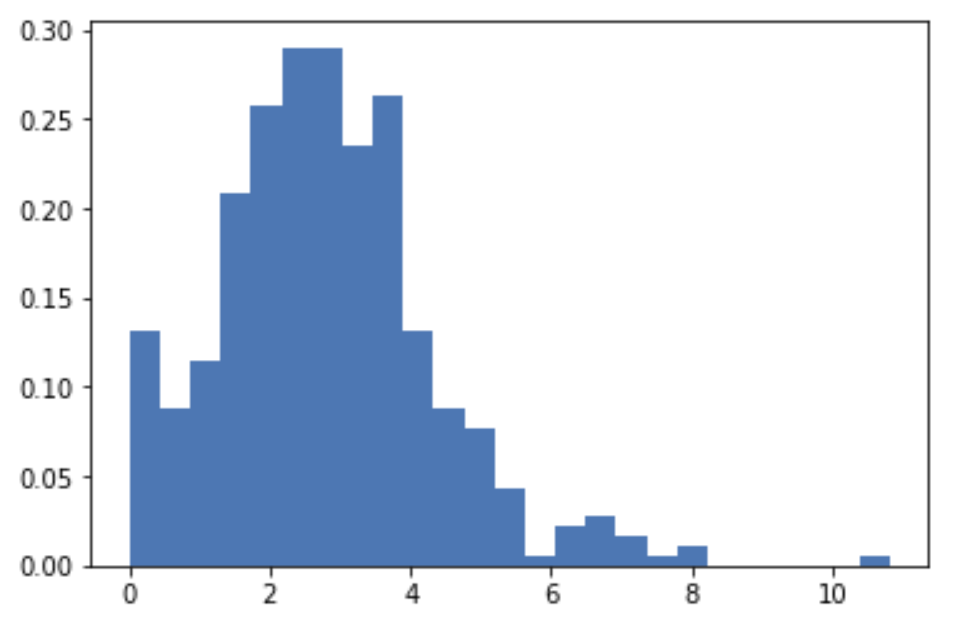这是4月2日全国各城市累计确诊新冠肺炎人数的直方图密度估计.
核密度估计
上节提到的直方图密度估计存在的问题是不光滑,核密度估计方法能够很好的解决这个问题.
定义
给定一组数据$x_1,x_2,\cdots,x_n$是取自于一个未知的连续分布$p(x)$,任意点$x$处的一种核密度估计定义为:
$\hat{p}(x)=\frac{1}{nh}\sum_{i=1}^nK \left ( \frac{x-x_i}{h} \right )$
其中$K(\cdot)$称为核函数(kernel function).$\hat{p}(x)$作为密度函数就需要保证非负和积分值为1,实际上只需要令核函数满足密度函数的定义即可:
$K(x)\ge0, \int K(x)\mathrm{d}x=1$
这时:
\begin{aligned} \int \hat{p}(x)\mathrm{d}x &= \int \frac{1}{n}\sum_{i=1}^n\frac{1}{h}K\left(\frac{x-x_i}{h} \right) \mathrm{d}x = \frac{1}{n}\sum_{i=1}^n \int\frac{1}{h}K\left( \frac{x-x_i}{h} \right)\mathrm{d}x \\ &= \frac{1}{n}\sum_{i=1}^n \int K(u)\mathrm{d}u=\frac{1}{n} \cdot n=1 \left(其中u=\frac{x-x_i}{h} \right). \end{aligned}
上式验证了$\hat{p}(x)$符合概率密度函数的定义,核密度估计中一个重要的部分就是核函数,常用的核函数有:

核函数名称
核函数$K(u)$

Parzen窗(均匀分布)
$\frac{1}{2}I(\lvert u\rvert \leq1)$

三角(Triangle)
$(1-\lvert u\rvert)I(\lvert u\rvert \leq1)$

Epanechikov
$\frac{3}{4}(1-u^2)I(\lvert u\rvert \leq1)$

四次(Quartic)
$\frac{15}{16}(1-u^2)I(\lvert u\rvert \leq1)$

三权(Triweight)
$\frac{35}{32}(1-u^2)^3I(\lvert u\rvert \leq1)$

高斯(Gauss)
$\frac{1}{\sqrt{2\pi}}\exp(-\frac{1}{2}u^2)$

余弦(Cosinus)
$\frac{\pi}{4}cos(\frac{\pi}{2}u)I(\lvert u\rvert \leq1)$

指数(Exponent)
$\exp(\lvert u \rvert)$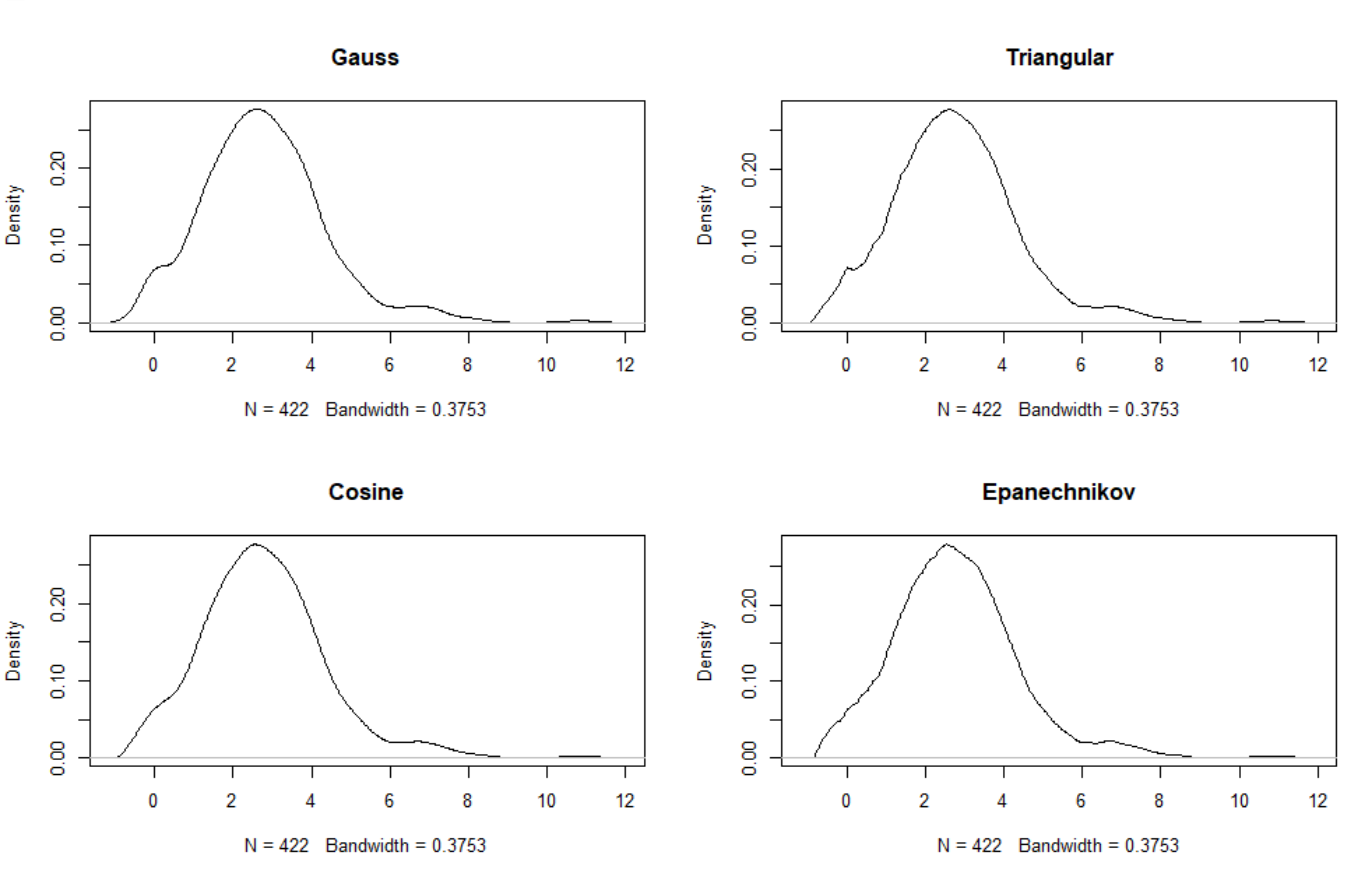可以看出,相同窗宽时不同的函数对数据密度曲线的估计情况差别不大,但如果调整窗宽后: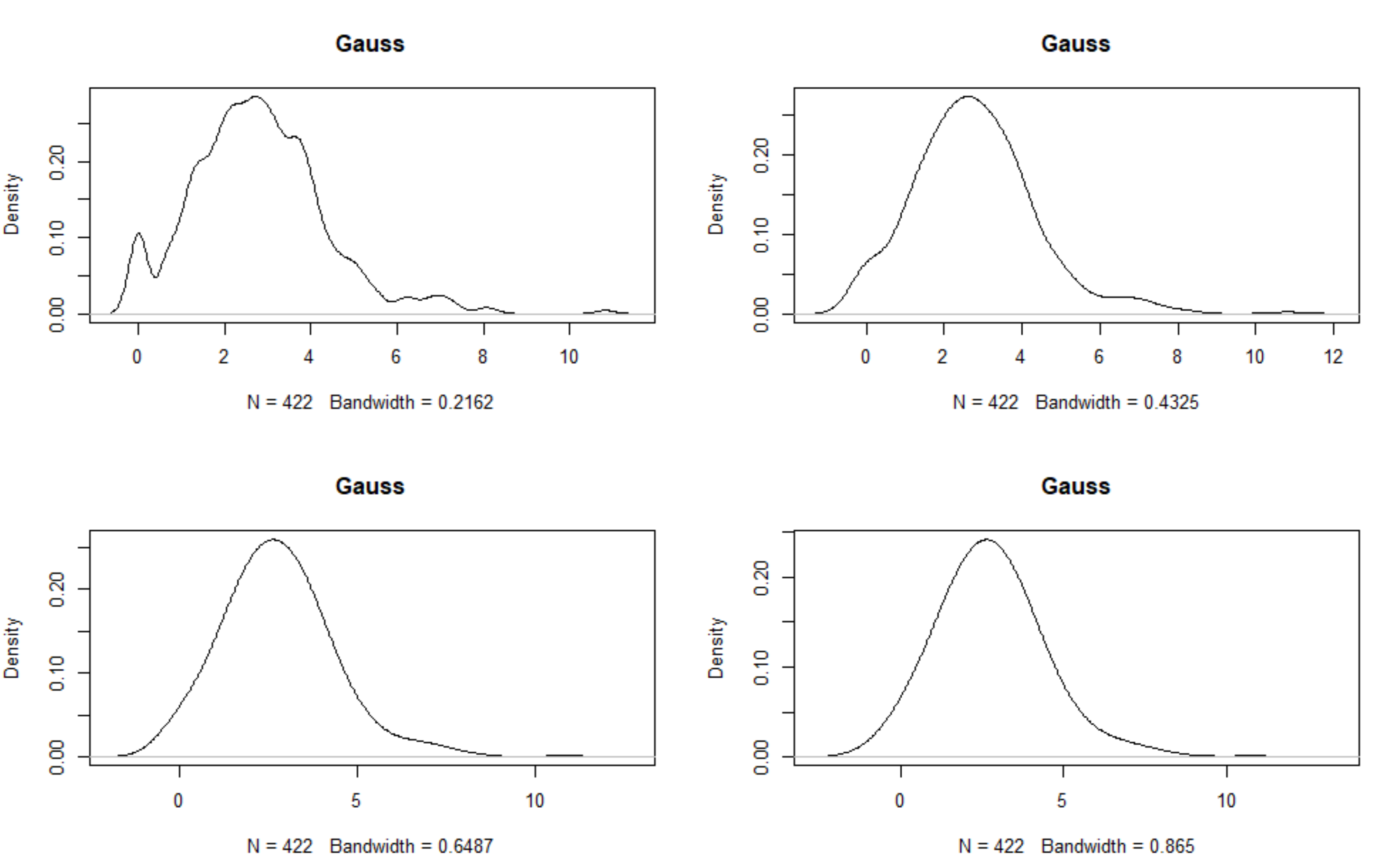同一核函数时,不断扩大核函数窗宽,数据的密度估计曲线越发接近于正态曲线,可见窗宽的选择相比较核函数的选择更为重要。那么如何选择合适的窗宽？理论上是从密度估计与真实密度之间的误差开始的。
窗宽选择
确定窗宽$h$使得估计的积分均方误差(mean integral square error,MISE)达到最小:
\begin{aligned} \mathrm{MISE}\{\hat{p}_h(x) \} &= E \left[ \int_{-\infty}^\infty \{ \hat{p_h}(x)-p(x)\}^2\mathrm{d}x \right] \\ &= \int_{-\infty}^\infty \mathrm{MSE}[\hat{p_h}(x)] \mathrm{d}x \\ &= \frac{1}{nh}\lVert K \rVert_2^2+\frac{h^4}{4}\mu_2(K)^2 \lVert p''\rVert_2^2+o(\frac{1}{nh}) +o(h^4) \end{aligned}
其中$\mu_2(K)=\int t^2K(t)\mathrm{d}t$,$t=(x-x_i)/h$.当$h\to\infty,nh\to\infty$时,可以得到渐进的MISE(AMISE)
$\mathrm{AMISE}=\{ \hat{p}(x)\}=\frac{1}{nh}\lVert K\rVert_2^2+\frac{h^4}{4}\mu_2(K)^2\lVert p''\rVert_2^2$
因此最优窗宽为:
$h_{opt}=\left( \frac{\lVert K\rVert_2^2}{\lVert p''\rVert_2^2\mu_2(K)^2n} \right)^{1/5} \backsim n^{1/5}$
通过上式可以知道,对于不同的核函数,我们可以得到相应的最优窗宽,例如高斯核函数时,可以得到$\mu_2=1,\int K(u)^2\mathrm{d}u=\int1/2\pi\exp(-u^2)\mathrm{d}u=\pi^{-1/2}$,那么最优窗宽就是$h_{opt}=1.06\sigma n^{-1/5}$.


展开全文统计学
• 原标题：核密度估计丨数析学院 核密度估计(kernel density estimation)是非参数统计检验中的重要方法之一，常用于估计未知的密度函数。本节将向大家介绍在 Python 中如何利用 Scikit-Learn 库中的相关工具，对数据集...
原标题：核密度估计丨数析学院核密度估计(kernel density estimation)是非参数统计检验中的重要方法之一，常用于估计未知的密度函数。本节将向大家介绍在 Python 中如何利用 Scikit-Learn 库中的相关工具，对数据集的样本分布进行核密度估计。导入可视化库：构建示例数据：可以看到，构建的数据为一维的随机数组，我们对其中后2/3的样本加上整数5。处理之后，其频率分布直方图如下所示：根据频率分布直方图的性质，所有柱形对应的频率之和为1:频率分布直方图是非参数统计中的重要可视化方法，为了演示方便，我们构造一组规模相对较小的数据集：在 Python 中，我们可以自定义直方图中的分组。下面我们用两种不同的分组方式，绘制两幅频率分布直方图，并将数据集中每个样本的值标注在横轴刻度下方。其中，右图为将默认的分组边界向右平移0.6之后的频率直方图结果：未完待续：课程内容较多，请复制链接通过电脑学习，获得最佳学习效果。http://datacademy.io/lesson/148更多课程和文章尽在微信号：「datartisan数据工匠」责任编辑：
展开全文• 核密度估计Kernel Density Estimation(KDE)概述密度估计的问题由给定样本集合求解随机变量的分布密度函数问题是概率统计学的基本问题之一。解决这一问题的方法包括参数估计和非参数估计。参数估计参数估计又可分为...
核密度估计Kernel Density Estimation(KDE)概述密度估计的问题由给定样本集合求解随机变量的分布密度函数问题是概率统计学的基本问题之一。解决这一问题的方法包括参数估计和非参数估计。参数估计参数估计又可分为参数回归分析和参数判别分析。在参数回归分析中，人们假定数据分布符合某种特定的性态，如线性、可化线性或指数性态等，然后在目标函数族中寻找特定的解，即确定回归模型中的未知参数。在参数判别分析中，人们需要假定作为判别依据的、随机取值的数据样本在各个可能的类别中都服从特定的分布。经验和理论说明，参数模型的这种基本假定与实际的物理模型之间常常存在较大的差距，这些方法并非总能取得令人满意的结果。非参数估计方法由于上述缺陷，Rosenblatt和Parzen提出了非参数估计方法，即核密度估计方法。由于核密度估计方法不利用有关数据分布的先验知识，对数据分布不附加任何假定，是一种从数据样本本身出发研究数据分布特征的方法，因而，在统计学理论和应用领域均受到高度的重视。核密度估计(kernel density estimation)是在概率论中用来估计未知的密度函数，属于非参数检验方法之一，由Rosenblatt (1955)和Emanuel Parzen(1962)提出，又名Parzen窗(Parzen window)。Ruppert和Cline基于数据集密度函数聚类算法提出修订的核密度估计方法。核密度估计在估计边界区域的时候会出现边界效应。[https://zh.wikipedia.org/zh-hans/核密度估计]因此，一句话概括，核密度估计Kernel Density Estimation(KDE)是在概率论中用来估计未知的密度函数，属于非参数检验方法之一。在密度函数估计中有一种方法是被广泛应用的——直方图。如下图中的第一和第二幅图(名为Histogram和Histogram, bins shifted)。直方图的特点是简单易懂，但缺点在于以下三个方面：密度函数是不平滑的；密度函数受子区间(即每个直方体)宽度影响很大，同样的原始数据如果取不同的子区间范围，那么展示的结果可能是完全不同的。如下图中的前两个图，第二个图只是在第一个图的基础上，划分区间增加了0.75，但展现出的密度函数却看起来差异很大；直方图最多只能展示2维数据，如果维度更多则无法有效展示。核密度估计有多种内核，图3(Tophat Kernl Density)为不平滑内核，图4(Gaussian Kernel Density,bandwidth=0.75)为平滑内核。在很多情况下，平滑内核(如高斯核密度估计，Gaussian Kernel Density)使用场景较多。虽然采用不同的核函数都可以获得一致性的结论(整体趋势和密度分布规律性基本一致)，但核密度函数也不是完美的。除了核算法的选择外，带宽(bandwidth)也会影响密度估计，过大或过小的带宽值都会影响估计结果。如上图中的最后三个图，名为Gaussian Kernel Density,bandwidth=0.75、Gaussian Kernel Density,bandwidth=0.25、Gaussian Kernel Density,bandwidth=0.55.核密度估计的应用场景股票、金融等风险预测：在单变量核密度估计的基础上，可以建立风险价值的预测模型。通过对核密度估计变异系数的加权处理，可以建立不同的风险价值的预测模型。密度估计中应用较多的算法是高斯混合模型以及基于近邻的核密度估计。高斯混合核密度估计模型更多会在聚类场景中应用。[核密度估计Kernel Density Estimation(KDE)]核密度分析可用于测量建筑密度、获取犯罪情况报告，以及发现对城镇或野生动物栖息地造成影响的道路或公共设施管线。可使用 population 字段根据要素的重要程度赋予某些要素比其他要素更大的权重，该字段还允许使用一个点表示多个观察对象。例如，一个地址可以表示一栋六单元的公寓，或者在确定总体犯罪率时可赋予某些罪行比其他罪行更大的权重。对于线要素，分车道高速公路可能比狭窄的土路产生更大的影响，高压线要比标准电线杆产生更大的影响。[ArcGIS中的介绍]热力图大家一定听说过，其实热力图就是核密度估计。总而言之，核密度就是用来估计密度的，如果你有一系列空间点数据，那么核密度估计往往是比较好的可视化方法核密度估计所谓核密度估计，就是采用平滑的峰值函数(“核”)来拟合观察到的数据点，从而对真实的概率分布曲线进行模拟。核密度估计(Kernel density estimation)，是一种用于估计概率密度函数的非参数方法，为独立同分布F的n个样本点，设其概率密度函数为f，核密度估计为以下：h>0为一个平滑参数，称作带宽(bandwidth)，也看到有人叫窗口。Kh(x) = 1/h K(x/h). 为缩放核函数(scaled Kernel)。核密度函数的原理比较简单，在我们知道某一事物的概率分布的情况下，如果某一个数在观察中出现了，我们可以认为这个数的概率密度很大，和这个数比较近的数的概率密度也会比较大，而那些离这个数远的数的概率密度会比较小。基于这种想法，针对观察中的第一个数，我们可以用K去拟合我们想象中的那个远小近大概率密度。对每一个观察数拟合出的多个概率密度分布函数，取平均。如果某些数是比较重要的，则可以取加权平均。需要说明的一点是，核密度的估计并不是找到真正的分布函数。Note: 核密度估计其实就是通过核函数(如高斯)将每个数据点的数据+带宽当作核函数的参数，得到N个核函数，再线性叠加就形成了核密度的估计函数，归一化后就是核密度概率密度函数了。以下面3个数据点的一维数据集为例：5, 10, 15绘制成直方图是这样的：                                                   而使用KDE则是：KDE核函数k(.)理论上，所有平滑的峰值函数均可作为KDE的核函数来使用，只要对归一化后的KDE而言(描绘在图上的是数据点出现的概率值)，该函数曲线下方的面积和等于1即可。只有一个数据点时，单个波峰下方的面积为1，存在多个数据点时，所有波峰下方的面积之和为1。概而言之，函数曲线需囊括所有可能出现的数据值的情况。常用的核函数有：矩形、Epanechnikov曲线、高斯曲线等。这些函数存在共同的特点：在数据点处为波峰；曲线下方面积为1。单个数据点(只有一个数据时)所对应的这些核函数矩形Epanechnikov曲线高斯曲线sklearn中实现的核函数sklearn核函数形式Gaussian kernel (kernel = 'gaussian')Tophat kernel (kernel = 'tophat')ifEpanechnikov kernel (kernel = 'epanechnikov')Exponential kernel (kernel = 'exponential')Linear kernel (kernel = 'linear')ifCosine kernel (kernel = 'cosine')if[Kernel Density Estimation¶]wekipedia上各种核函数的图形均匀核函数 k(x)=1/2,-1≤x≤1 加入带宽h后： kh(x)=1/(2h),-h≤x≤h三角核函数 k(x)=1-|x|,-1≤x≤1 加入带宽h后： kh(x)=(h-|x|)/h^2,-h≤x≤h伽马核函数 kxi(x)=[x^(α-1)exp{-xα/xi}]/[(xi/α)^α.Γ(α)]高斯核函数K(x,xc)=exp(-||x-xc||^2/(2*σ)^2)其中xc为核函数中心,σ为函数的宽度参数[https://zh.wikipedia.org/zh-hans/%E6%A0%B8%E5%AF%86%E5%BA%A6%E4%BC%B0%E8%AE%A1]不同内核的比较Epanechnikov 内核在均方误差意义下是最优的，效率损失也很小。由于高斯内核方便的数学性质，也经常使用 K(x)= ϕ(x)，ϕ(x)为标准正态概率密度函数。对于多个数据点的KDE曲线：由于相邻波峰之间会发生波形合成，因此最终所形成的曲线形状与选择的核函数关系并不密切。考虑到函数在波形合成计算上的易用性，一般使用高斯曲线(正态分布曲线)作为KDE的核函数。KDE算法：索引树lz发现sklearn算法实现中有一个参数是算法项，如algorithm='auto'，想了一下是为了加速。KDE的概率密度函数公式得到后有了上述公式之后，只需遍历输出图像的每一个点，计算其核密度估计值即可。但是稍微想一下就发现这个程序太冗余了，如果有很多点(n很大)，并且输出图像很大，那么每一个像素都需要进行n个累积的加法运算，并且大部分都是+0(因为一般来说，一个点附近的点不会很多，远远小于n，其余大部分点与这个像素的距离都大于r)，这样就造成了冗余计算。解决方案当然也非常简单，就是建立一个索引，然后在计算某个像素的核密度估计值时利用索引搜索出附近的点，然后累积这些点的核函数即可。如Dotspatial自带了多种空间索引，有R树，R*树，KD树等；sklearn自带了kd tree, ball tree等等。如果只需找出附近的点，对索引要求不高，任意一个索引都能使用。[空间点云核密度估计算法的实现-以Dotspatial为基础GIS库]KDE带宽h如何选定核函数的“方差”呢？这其实是由带宽h来决定，不同的带宽下的核函数估计结果差异很大。带宽反映了KDE曲线整体的平坦程度，也即观察到的数据点在KDE曲线形成过程中所占的比重。带宽越大，观察到的数据点在最终形成的曲线形状中所占比重越小，KDE整体曲线就越平坦；带宽越小，观察到的数据点在最终形成的曲线形状中所占比重越大，KDE整体曲线就越陡峭。还是以上面3个数据点的一维数据集为例，如果增加带宽，那么生成的KDE曲线就会变平坦：如果进一步增加带宽，那么KDE曲线在变平坦的同时，还会发生波形合成：相反，如果减少带宽，那么KDE曲线就会变得更加陡峭：从数学上来说，对于数据点Xi，如果带宽为h，那么在Xi处所形成的曲线函数为(其中K为核函数)：在上面的函数中，K函数内部的h分母用于调整KDE曲线的宽幅，而K函数外部的h分母则用于保证曲线下方的面积符合KDE的规则(KDE曲线下方面积和为1)。带宽的选择带宽的选择很大程度上取决于主观判断：如果认为真实的概率分布曲线是比较平坦的，那么就选择较大的带宽；相反，如果认为真实的概率分布曲线是比较陡峭的，那么就选择较小的带宽。带宽计算好像也有相应的方法，如R语言中计算带宽时，默认采用”nrd0″方法。如何选择h？显然是选择可以使误差最小的。下面用平均积分平方误差(mean intergrated squared error)的大小来衡量h的优劣。在weak assumptions下，MISE (h) =AMISE(h) + o(1/(nh) + h4) ，其中AMISE为渐进的MISE。而AMISE有，，其中，，为了使MISE(h)最小，则转化为求极点问题，当核函数确定之后，h公式里的R、m、f''都可以确定下来，有(hAMISE ~ n−1/5)，AMISE(h) = O(n−4/5)。如果带宽不是固定的，其变化取决于估计的位置(balloon  estimator)或样本点(逐点估计pointwise estimator)，由此可以产产生一个非常强大的方法称为自适应或可变带宽核密度估计。[核密度估计(Kernel density estimation)]在选择合适的核函数及带宽后，KDE可以模拟真实的概率分布曲线，并得到平滑而漂亮的结果。以近200个点的CPU使用率为例，使用KDE绘制的结果为：[一维数据可视化：核密度估计(Kernel Density Estimates)]核密度估计的实现Python中KDE的实现：sklearn[sklearn.neighbors.KernelDensity(bandwidth=1.0, algorithm='auto', kernel='gaussian', metric='euclidean', atol=0, rtol=0, breadth_first=True, leaf_size=40, metric_params=None)from sklearn.neighbors import kdeimport numpy as npX = np.array([[-1, -1], [-2, -1], [-3, -2], [1, 1], [2, 1], [3, 2]])kde = kde.KernelDensity(kernel='gaussian', bandwidth=0.2).fit(X)print(kde.score_samples(X))print(np.exp(kde.score_samples(X)))[-0.41075698 -0.41075698 -0.41076071 -0.41075698 -0.41075698 -0.41076071][ 0.66314807  0.66314807  0.6631456   0.66314807  0.66314807  0.6631456 ]score_samples(X)Evaluate the density model on the data.Parameters:X : array_like, shape (n_samples, n_features)kde.score_samples(X)返回的是点x对应概率的log值，要使用exp求指数还原。Note: 还原后的所有点的概率和范围是[0, 无穷大]，只是说一维数据线下面的面积或者二维数据面下面的体积和为1。[Density Estimation¶][sklearn.neighbors.KernelDensity¶]spark中KDE的实现MLlib中，仅仅支持以高斯核做核密度估计。R中KDE的实现在R语言中，KDE的绘制是通过density()函数来实现的 — 通过density()函数计算得到KDE模型，然后再使用plot()函数对KDE曲线进行绘制：x plot(density(x))出于兼容性上的考虑，R语言中density()函数在计算带宽时，默认采用”nrd0″方法。不过，根据R语言的帮助文档，带宽参数bw应该显式声明为其它更合适的方法，比如”SJ”：plot(density(x, bw="SJ"))对于调整带宽，除了修改bw参数，还可以通过设定adjust参数来进行扩大或缩小：plot(density(x, bw="SJ", adjust=1.5))在上面的例子中，最终使用的带宽将是采用”SJ”方法计算得到的带宽的1.5倍。adjust参数的默认值为1，也即既不扩大、也不缩小。至于核函数，density()默认采用高斯曲线。可以通过设定kernel参数来更改核函数。比如：plot(density(x, bw="SJ", kernel="epanechnikov"))density()函数接受以下7个核函数选项：gaussian。高斯曲线，默认选项。在数据点处模拟正态分布。epanechnikov。Epanechnikov曲线。rectangular。矩形核函数。triangular。三角形核函数。biweight。cosine。余弦曲线。optcosine。
展开全文• ## 非参数估计：核密度估计KDE

万次阅读 多人点赞 2016-12-14 11:38:46
http://blog.csdn.net/pipisorry/article/details/53635895核密度估计Kernel Density Estimation(KDE)概述密度估计的问题由给定样本集合求解随机变量的分布密度函数问题是概率统计学的基本问题之一。解决这一问题的...
http://blog.csdn.net/pipisorry/article/details/53635895核密度估计Kernel Density Estimation(KDE)概述密度估计的问题由给定样本集合求解随机变量的分布密度函数问题是概率统计学的基本问题之一。解决这一问题的方法包括参数估计和非参数估计。参数估计参数估计又可分为参数回归分析和参数判别分析。在参数回归分析中，人们假定数据分布符合某种特定的性态，如线性、可化线性或指数性态等，然后在目标函数族中寻找特定的解，即确定回归模型中的未知参数。在参数判别分析中，人们需要假定作为判别依据的、随机取值的数据样本在各个可能的类别中都服从特定的分布。经验和理论说明，参数模型的这种基本假定与实际的物理模型之间常常存在较大的差距，这些方法并非总能取得令人满意的结果。[参数估计：最大似然估计MLE][参数估计：文本分析的参数估计方法]非参数估计方法由于上述缺陷，Rosenblatt和Parzen提出了非参数估计方法，即核密度估计方法。由于核密度估计方法不利用有关数据分布的先验知识，对数据分布不附加任何假定，是一种从数据样本本身出发研究数据分布特征的方法，因而，在统计学理论和应用领域均受到高度的重视。核密度估计（kernel density estimation）是在概率论中用来估计未知的密度函数，属于非参数检验方法之一，由Rosenblatt (1955)和Emanuel Parzen(1962)提出，又名Parzen窗（Parzen window）。Ruppert和Cline基于数据集密度函数聚类算法提出修订的核密度估计方法。核密度估计在估计边界区域的时候会出现边界效应。[https://zh.wikipedia.org/zh-hans/核密度估计]因此，一句话概括，核密度估计Kernel Density Estimation(KDE)是在概率论中用来估计未知的密度函数，属于非参数检验方法之一。在密度函数估计中有一种方法是被广泛应用的——直方图。如下图中的第一和第二幅图（名为Histogram和Histogram, bins shifted）。直方图的特点是简单易懂，但缺点在于以下三个方面：密度函数是不平滑的；密度函数受子区间（即每个直方体）宽度影响很大，同样的原始数据如果取不同的子区间范围，那么展示的结果可能是完全不同的。如下图中的前两个图，第二个图只是在第一个图的基础上，划分区间增加了0.75，但展现出的密度函数却看起来差异很大；直方图最多只能展示2维数据，如果维度更多则无法有效展示。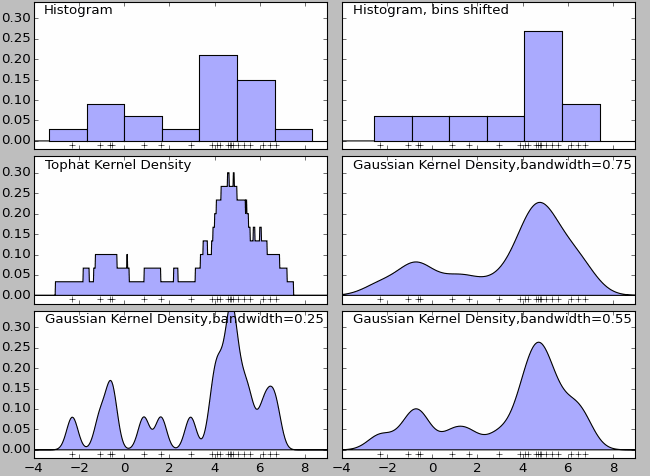核密度估计有多种内核，图3（Tophat Kernl Density）为不平滑内核，图4（Gaussian Kernel Density,bandwidth=0.75）为平滑内核。在很多情况下，平滑内核（如高斯核密度估计，Gaussian Kernel Density）使用场景较多。虽然采用不同的核函数都可以获得一致性的结论（整体趋势和密度分布规律性基本一致），但核密度函数也不是完美的。除了核算法的选择外，带宽（bandwidth）也会影响密度估计，过大或过小的带宽值都会影响估计结果。如上图中的最后三个图，名为Gaussian Kernel Density,bandwidth=0.75、Gaussian Kernel Density,bandwidth=0.25、Gaussian Kernel Density,bandwidth=0.55.核密度估计的应用场景股票、金融等风险预测：在单变量核密度估计的基础上，可以建立风险价值的预测模型。通过对核密度估计变异系数的加权处理，可以建立不同的风险价值的预测模型。密度估计中应用较多的算法是高斯混合模型以及基于近邻的核密度估计。高斯混合核密度估计模型更多会在聚类场景中应用。[核密度估计Kernel Density Estimation(KDE)]核密度分析可用于测量建筑密度、获取犯罪情况报告，以及发现对城镇或野生动物栖息地造成影响的道路或公共设施管线。可使用 population 字段根据要素的重要程度赋予某些要素比其他要素更大的权重，该字段还允许使用一个点表示多个观察对象。例如，一个地址可以表示一栋六单元的公寓，或者在确定总体犯罪率时可赋予某些罪行比其他罪行更大的权重。对于线要素，分车道高速公路可能比狭窄的土路产生更大的影响，高压线要比标准电线杆产生更大的影响。[ArcGIS中的介绍]热力图大家一定听说过，其实热力图就是核密度估计。总而言之，核密度就是用来估计密度的，如果你有一系列空间点数据，那么核密度估计往往是比较好的可视化方法皮皮blog核密度估计所谓核密度估计，就是采用平滑的峰值函数(“核”)来拟合观察到的数据点，从而对真实的概率分布曲线进行模拟。核密度估计（Kernel density estimation），是一种用于估计概率密度函数的非参数方法，为独立同分布F的n个样本点，设其概率密度函数为f，核密度估计为以下：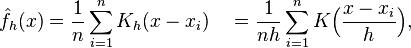K(.)为核函数（非负、积分为1，符合概率密度性质，并且均值为0）。有很多种核函数，uniform,triangular, biweight, triweight, Epanechnikov,normal等。h>0为一个平滑参数，称作带宽(bandwidth)，也看到有人叫窗口。Kh(x) = 1/h K(x/h). 为缩放核函数(scaled Kernel)。核密度函数的原理比较简单，在我们知道某一事物的概率分布的情况下，如果某一个数在观察中出现了，我们可以认为这个数的概率密度很大，和这个数比较近的数的概率密度也会比较大，而那些离这个数远的数的概率密度会比较小。基于这种想法，针对观察中的第一个数，我们可以用K去拟合我们想象中的那个远小近大概率密度。对每一个观察数拟合出的多个概率密度分布函数，取平均。如果某些数是比较重要的，则可以取加权平均。需要说明的一点是，核密度的估计并不是找到真正的分布函数。Note: 核密度估计其实就是通过核函数（如高斯）将每个数据点的数据+带宽当作核函数的参数，得到N个核函数，再线性叠加就形成了核密度的估计函数，归一化后就是核密度概率密度函数了。以下面3个数据点的一维数据集为例：5, 10, 15绘制成直方图是这样的：                                                   而使用KDE则是：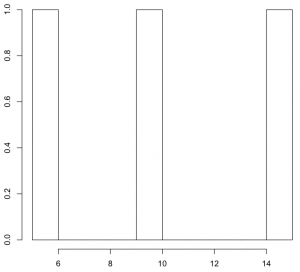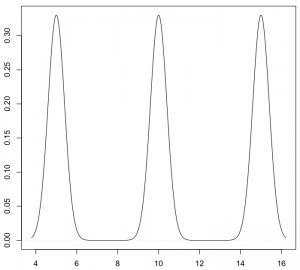KDE核函数k(.)理论上，所有平滑的峰值函数均可作为KDE的核函数来使用，只要对归一化后的KDE而言(描绘在图上的是数据点出现的概率值)，该函数曲线下方的面积和等于1即可。 只有一个数据点时，单个波峰下方的面积为1，存在多个数据点时，所有波峰下方的面积之和为1。概而言之，函数曲线需囊括所有可能出现的数据值的情况。常用的核函数有：矩形、Epanechnikov曲线、高斯曲线等。这些函数存在共同的特点：在数据点处为波峰；曲线下方面积为1。单个数据点（只有一个数据时）所对应的这些核函数矩形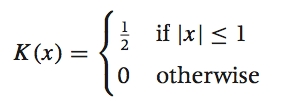Epanechnikov曲线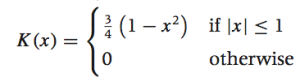高斯曲线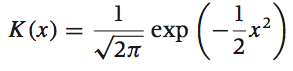[概率论：高斯/正态分布                    ]sklearn中实现的核函数sklearn核函数形式Gaussian kernel (kernel = 'gaussian')Tophat kernel (kernel = 'tophat')ifEpanechnikov kernel (kernel = 'epanechnikov')Exponential kernel (kernel = 'exponential')Linear kernel (kernel = 'linear')ifCosine kernel (kernel = 'cosine')if[Kernel Density Estimation¶]wekipedia上各种核函数的图形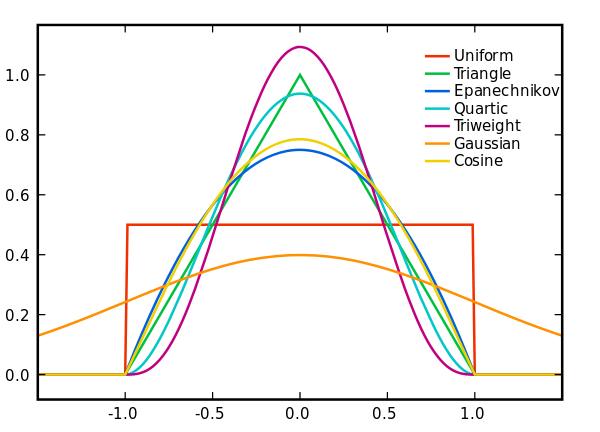均匀核函数 k(x)=1/2,-1≤x≤1 加入带宽h后： kh(x)=1/(2h),-h≤x≤h三角核函数 k(x)=1-|x|,-1≤x≤1 加入带宽h后： kh(x)=(h-|x|)/h^2,-h≤x≤h伽马核函数 kxi(x)=[x^(α-1)exp{-xα/xi}]/[(xi/α)^α.Γ(α)]高斯核函数K(x,xc)=exp(-||x-xc||^2/(2*σ)^2)其中xc为核函数中心,σ为函数的宽度参数[https://zh.wikipedia.org/zh-hans/%E6%A0%B8%E5%AF%86%E5%BA%A6%E4%BC%B0%E8%AE%A1]不同内核的比较 Epanechnikov 内核在均方误差意义下是最优的，效率损失也很小。由于高斯内核方便的数学性质，也经常使用 K(x)= ϕ(x)，ϕ(x)为标准正态概率密度函数。对于多个数据点的KDE曲线：由于相邻波峰之间会发生波形合成，因此最终所形成的曲线形状与选择的核函数关系并不密切。考虑到函数在波形合成计算上的易用性，一般使用高斯曲线(正态分布曲线)作为KDE的核函数。KDE算法：索引树lz发现sklearn算法实现中有一个参数是算法项，如algorithm='auto'，想了一下是为了加速。KDE的概率密度函数公式得到后有了上述公式之后，只需遍历输出图像的每一个点，计算其核密度估计值即可。但是稍微想一下就发现这个程序太冗余了，如果有很多点（n很大），并且输出图像很大，那么每一个像素都需要进行n个累积的加法运算，并且大部分都是+0（因为一般来说，一个点附近的点不会很多，远远小于n，其余大部分点与这个像素的距离都大于r），这样就造成了冗余计算。解决方案当然也非常简单，就是建立一个索引，然后在计算某个像素的核密度估计值时利用索引搜索出附近的点，然后累积这些点的核函数即可。如Dotspatial自带了多种空间索引，有R树，R*树，KD树等；sklearn自带了kd tree, ball tree等等。如果只需找出附近的点，对索引要求不高，任意一个索引都能使用。[空间点云核密度估计算法的实现-以Dotspatial为基础GIS库]KDE带宽h如何选定核函数的“方差”呢？这其实是由带宽h来决定，不同的带宽下的核函数估计结果差异很大。带宽反映了KDE曲线整体的平坦程度，也即观察到的数据点在KDE曲线形成过程中所占的比重。带宽越大，观察到的数据点在最终形成的曲线形状中所占比重越小，KDE整体曲线就越平坦；带宽越小，观察到的数据点在最终形成的曲线形状中所占比重越大，KDE整体曲线就越陡峭。还是以上面3个数据点的一维数据集为例，如果增加带宽，那么生成的KDE曲线就会变平坦：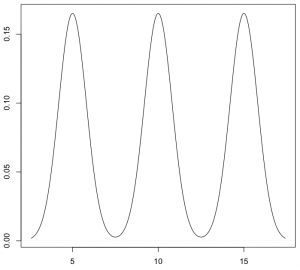如果进一步增加带宽，那么KDE曲线在变平坦的同时，还会发生波形合成：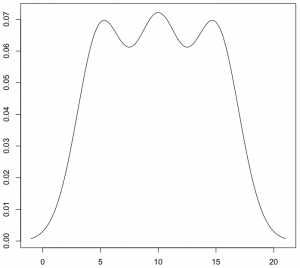相反，如果减少带宽，那么KDE曲线就会变得更加陡峭：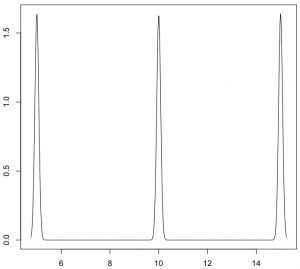从数学上来说，对于数据点Xi，如果带宽为h，那么在Xi处所形成的曲线函数为(其中K为核函数)：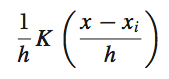在上面的函数中，K函数内部的h分母用于调整KDE曲线的宽幅，而K函数外部的h分母则用于保证曲线下方的面积符合KDE的规则(KDE曲线下方面积和为1)。带宽的选择带宽的选择很大程度上取决于主观判断：如果认为真实的概率分布曲线是比较平坦的，那么就选择较大的带宽；相反，如果认为真实的概率分布曲线是比较陡峭的，那么就选择较小的带宽。带宽计算好像也有相应的方法，如R语言中计算带宽时，默认采用”nrd0″方法。如何选择h？显然是选择可以使误差最小的。下面用平均积分平方误差（mean intergrated squared error）的大小来衡量h的优劣。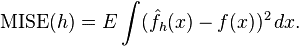在weak assumptions下，MISE (h) =AMISE(h) + o(1/(nh) + h4) ，其中AMISE为渐进的MISE。而AMISE有，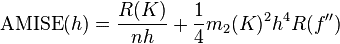，其中，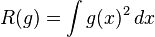，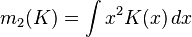为了使MISE(h)最小，则转化为求极点问题，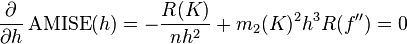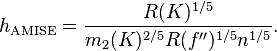当核函数确定之后，h公式里的R、m、f''都可以确定下来，有（hAMISE ~ n−1/5），AMISE(h) = O(n−4/5)。        如果带宽不是固定的，其变化取决于估计的位置（balloon  estimator）或样本点（逐点估计pointwise estimator），由此可以产产生一个非常强大的方法称为自适应或可变带宽核密度估计。[核密度估计(Kernel density estimation)                    ]在选择合适的核函数及带宽后，KDE可以模拟真实的概率分布曲线，并得到平滑而漂亮的结果。以近200个点的CPU使用率为例，使用KDE绘制的结果为：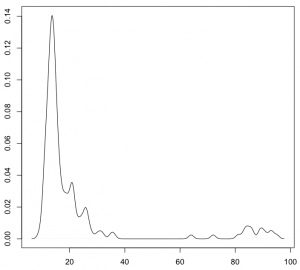[一维数据可视化：核密度估计(Kernel Density Estimates)]皮皮blog核密度估计的实现Python中KDE的实现：sklearn[sklearn.neighbors.KernelDensity(bandwidth=1.0, algorithm='auto', kernel='gaussian', metric='euclidean', atol=0, rtol=0, breadth_first=True, leaf_size=40, metric_params=None)from sklearn.neighbors import kde
import numpy as np

X = np.array([[-1, -1], [-2, -1], [-3, -2], [1, 1], [2, 1], [3, 2]])
kde = kde.KernelDensity(kernel='gaussian', bandwidth=0.2).fit(X)
print(kde.score_samples(X))
print(np.exp(kde.score_samples(X)))[-0.41075698 -0.41075698 -0.41076071 -0.41075698 -0.41075698 -0.41076071][ 0.66314807  0.66314807  0.6631456   0.66314807  0.66314807  0.6631456 ]score_samples(X)Evaluate the density model on the data.Parameters:X : array_like, shape (n_samples, n_features)kde.score_samples(X)返回的是点x对应概率的log值，要使用exp求指数还原。Note: 还原后的所有点的概率和范围是[0, 无穷大]，只是说一维数据线下面的面积或者二维数据面下面的体积和为1。[Density Estimation¶][sklearn.neighbors.KernelDensity¶]spark中KDE的实现MLlib中，仅仅支持以高斯核做核密度估计。[核密度估计]R中KDE的实现在R语言中，KDE的绘制是通过density()函数来实现的 — 通过density()函数计算得到KDE模型，然后再使用plot()函数对KDE曲线进行绘制：x <- c(5, 10, 15)plot(density(x))出于兼容性上的考虑，R语言中density()函数在计算带宽时，默认采用”nrd0″方法。不过，根据R语言的帮助文档，带宽参数bw应该显式声明为其它更合适的方法，比如”SJ”：plot(density(x, bw="SJ"))对于调整带宽，除了修改bw参数，还可以通过设定adjust参数来进行扩大或缩小：plot(density(x, bw="SJ", adjust=1.5))在上面的例子中，最终使用的带宽将是采用”SJ”方法计算得到的带宽的1.5倍。adjust参数的默认值为1，也即既不扩大、也不缩小。至于核函数，density()默认采用高斯曲线。可以通过设定kernel参数来更改核函数。比如：plot(density(x, bw="SJ", kernel="epanechnikov"))density()函数接受以下7个核函数选项：    gaussian。高斯曲线，默认选项。在数据点处模拟正态分布。    epanechnikov。Epanechnikov曲线。    rectangular。矩形核函数。    triangular。三角形核函数。    biweight。    cosine。余弦曲线。    optcosine。from: http://blog.csdn.net/pipisorry/article/details/53635895ref: [有边界区间上的核密度估计]
展开全文kde 非参数估计
• 核密度估计参考教案： 根据从一个总体中抽出的样本取估计总体分布的密度函数，在应用上有重要的意义。 关于密度函数的参数估计就是在假定该随即变量的密度函数的形式已知的前提下，对密度函数的参数进行估计。
• matlab核密度估计程序，用于数据的预测，风险控制，预期收益等
• kde核密度估计，非参数估计的一种，使用高斯核函数进行概率密度估计，应用于独立成分分析确定控制限等过程
• 我想绘制核密度估计的等高线图,其中KDE集成在每个等高线图填充区域内.举个例子,假设我计算了2D数据的KDE：data = np.random.multivariate_normal((0, 0), [[1, 1], [2, 0.7]], 100)x = data[:, 0]y = data[:, 1]xmin...
• 1.核密度估计图。import matplotlib.pylab as pltimport seaborn as snsdf = sns.load_dataset('iris')p1=sns.kdeplot(df['sepal_width'])plt.show()import matplotlib.pylab as pltimport seaborn as snsdf = sns....
• 我想绘制核密度估计的等高线图,其中KDE集成在每个等高线图填充区域内.举个例子,假设我计算了2D数据的KDE：data = np.random.multivariate_normal((0, 0), [[1, 1], [2, 0.7]], 100)x = data[:, 0]y = data[:, 1]xmin...
• ## 多维核密度估计

千次阅读 2020-04-17 00:27:35
多元核密度估计 承接上一篇文章,接着讨论多维随机变量情况下的核密度估计。 定义 给定一组样本X={x1,x2,⋯ ,xn}\bm{X}=\{ \bm{x}_1,\bm{x}_2,\cdots,\bm{x}_n \}X={x1​,x2​,⋯,xn​}且都是ddd维的向量,并且取自同...
• 东华大学机器学习核密度估计大作业 第一部分是一个三维的彩色KDE估计图（最好用MATLAB画）；第二部分是测试图片的运动目标二值图像检测结果（运动员用白色像素，背景用黑色）
•研究论文
• 非参数加权核密度估计的相合性和渐近正态性，魏玉华，夏乐天，本文在传统的核密度估计理论的基础上，提出了加权核密度估计，并得出在一定的条件下，加权核密度估计与传统的核密度估计一样，也
• 这个资源是matlab实现的基于KDE（核密度估计）的行人检测，包含代码和数据·库。清清楚楚，明明白白，注释易懂。本人课堂大作业的完整版。
• R语言与核密度估计非参数统计Tag内容描述：1、R语言与非参数统计(核密度估计),核密度估计是在概率论中用来估计未知的密度函数，属于非参数检验方法之一，由Rosenblatt (1955)和Emanuel Parzen(1962)提出，又名Parzen...
• 提出了一种基于核密度估计相似性测度的立体匹配方法。将匹配基元中对应像素点的颜色差值在差值特征空间中进行核密度估计,并以此作为相似性测度。在此基础上建立全局能量函数,并采用改进的置信度传播算法进行视差图的...
• 最近看论文，发现一个很不错的概率密度估计方法。在此小记一下。 先来看看准备知识。 密度估计经常在统计学中作为一种使用有限的样本来估计其概率密度函数的方法。 我们在研究随机变量的过程中，随机变量的概率...统计学 非参数估计 自适应带宽
•研究论文...